GeeksforGeeks App
Open AppBrowser
Continue

# Direction Sense test

Direction Sense is an important topic of Logical Reasoning. On this topic we will learn how to detect the direction according to the given situations.
Basically , there are four major directions – East (E) , West (W), North (N) and South (S)
Directions are shown in the given figure.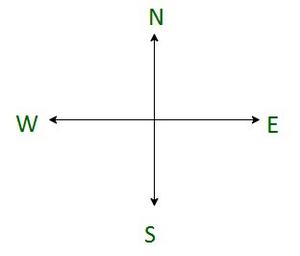An image showing the 4 Directions

Also, there are four cardinal directions, which are North-East (N-E), North-West (N-W), South-East (S-E), and South-West (S-W).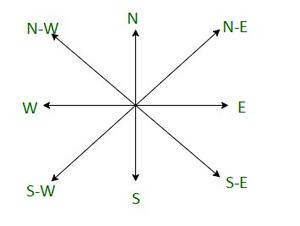An image showing 4 Cardinal directions

To solve the direction sense test questions, you need to make a sketch of the data provided and according to that sketch you will easily get to the answer.

In the morning, as the sun rises from the east, so the shadow of any objects formed is in the west direction.
At noon, or 12 pm, when the sun is exactly on top , no shadow will form.
In the evening , as the sun sets in the West , so the shadow  of any objects formed is in the East.
Keeping these directions in mind, there are some types of questions which come into the exams, which we will discuss one by one.

## Direction Sense Questions and Answers

### Q1: Ram starts from a point A walks 10 km north, then turns right and walks for 7 km, then turns right again and walks for another 10 km.  And reaches point B. How far is Ram from the starting point?

Solution:
Now, according to the question, and the directions in mind,So, from the above image it is clear that ram is 7 km away from the starting point.

### Q2: Sam started walking from point A towards East and walked for 6 km, then he turned to the left and walked for 8 km to reach point B. How far was he from the starting point?

Solution:
Now, according to the question,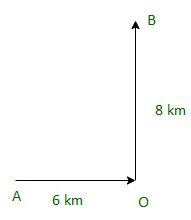Now, to the Distance between A and B = √( 62 + 82)          (By Pythagoras’ theorem)

= √100

= 10 km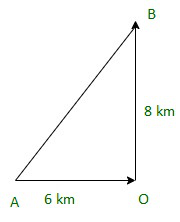Therefore, the distance from the starting point is 10 km.

### Q3: One morning after sunrise, Mahesh was standing facing a pole. The shadow of the pole fell exactly to his right. To which direction was he facing?

Solution:
The sun rises in the East (E) in the morning.
As the shadow of Mahesh falls to his right.
So he must be facing South.
Hence, the answer is the south.

### Q4: M is in the East of N, which is in the North of S. If P is in the South of S, then in which direction of M, is P?

Solution:
Let us understand through the diagram , which will clear the question more.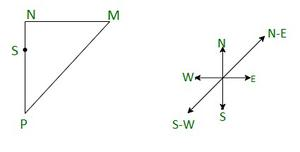So, P is south-west of M.

### Q5: You are facing south. You turn right and walk 20 m , then you turn right and walk 10m. Then turn left and walk for 10 m. then turn right and walk for 20 m. Then you turn right again and walk 60 m. In which direction do you in from the starting point?

Solution:
First we have to make a sketch of all the given data and then we will analyze it to get the answer or to know the direction,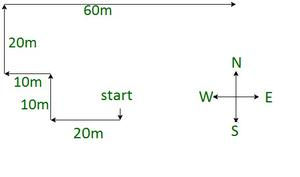we start facing south, and after that follow the paths given in question above,
Now, we have to find a direction from the starting point.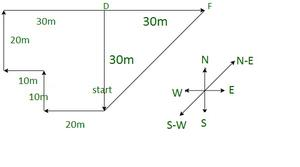Here , we will also find the distance from start to F , distance = √(302 + 302 ) = 30√2.
and the direction is N-E ( north east).

My Personal Notes arrow_drop_up